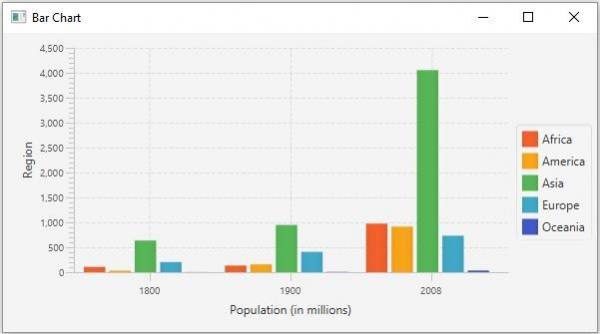# How to create a bar chart using JavaFX?

The bar chart accepts a series of data points (x, y) as input values, and creates bars representing their values. Typically, these charts are used to represent the value of a category. Depending on the axis of the category the bars of a bar chart can be vertical or horizontal. In JavaFX, you can create a bar chart by instantiating the javafx.scene.chart.BarChart class.

While instantiating this class you must pass the two objects of the Axis class representing the x and y-axis (as parameters of the constructor). Since the Axis class is abstract you need to pass objects of its concrete subclasses, NumberAxis (for numerical values) or, CategoryAxis (String values).

Once you create the axes you can set labels to them using the setLabel() method.

## Setting data

The XYChart.Series represents the series of data items. You can create a series of points for the bars by instantiating this class. This class contains an observable list that holds all the points in the series.

The XYChart.Data represents a specific data point in the x-y plane. To create a point, you need to instantiate this class by passing the x and y values of the particular point.

Therefore, to create data for a bar −

• Create a required number of points by instantiating the XYChart.Data class.

• Create a series by instantiating the XYChart.Series class.

• Get the observable list of the XYChart.Series class using the getData() method.

• Add the created data series to the area chart as −

barChart.getData().add(series);

## Example

import javafx.application.Application;
import javafx.geometry.Side;
import javafx.scene.Scene;
import javafx.scene.chart.BarChart;
import javafx.scene.chart.CategoryAxis;
import javafx.stage.Stage;
import javafx.scene.chart.NumberAxis;
import javafx.scene.chart.XYChart;
import javafx.scene.layout.StackPane;
public class BarChartExample extends Application {
public void start(Stage stage) {
//Creating X and Y axes
CategoryAxis xAxis = new CategoryAxis();
NumberAxis yAxis = new NumberAxis();
yAxis.setLabel("Region");
xAxis.setLabel("Population (in millions)");
//Creating a Bar chart
BarChart barChart = new BarChart<>(xAxis, yAxis);
//Preparing data for the bar chart
XYChart.Series series1 = new XYChart.Series();
series1.setName("Africa");
XYChart.Series series2 = new XYChart.Series();
series2.setName("America");
XYChart.Series series3 = new XYChart.Series();
series3.setName("Asia");
XYChart.Series series4 = new XYChart.Series();
series4.setName("Europe");
XYChart.Series series5 = new XYChart.Series();
series5.setName("Oceania");
//Setting the data to bar chart
//Setting the legend on the top
barChart.setLegendSide(Side.RIGHT);
//Creating a stack pane to hold the chart
StackPane pane = new StackPane(barChart);
//Setting the Scene
Scene scene = new Scene(pane, 595, 300);
stage.setTitle("Bar Chart");
stage.setScene(scene);
stage.show();
}
public static void main(String args[]){
launch(args);
}
}

## Output